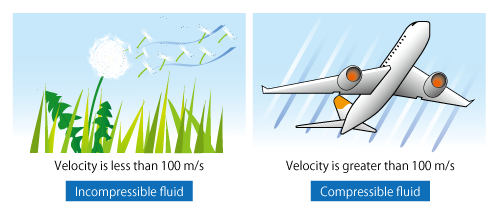# Want to Know More! Basics of Thermo-Fluid Analysis 16: Chapter 3 Flow 3.3.2 Compressibility and incompressibility (1)

#### 3.3.2 Compressibility and incompressibility (1)

The volume of real fluids changes when they are expanded or compressed by an external force or the change of pressure or temperature. The property of volume change is called compressibility and a fluid whose volume changes is called compressible fluid.

On the other hand, an incompressible fluid is a fluid which is not compressed or expanded, and its volume is always constant. In reality, a rigorous incompressible fluid does not exist. However, when a flow is less affected by compressibility, as a flow of air or water around us, the flow can be considered as an incompressible fluid flow.

The magnitude of compressibility effect can be judged with flow velocity. For air, when flow velocity is 100 m/s or less, the air is treated as an incompressible fluid, and when the velocity is greater than 100 m/s, the air is treated as compressible fluid. As shown in Figure 3.19, an air flow with low velocity like breeze is an incompressible fluid while an air with high velocity like a flow around an airplane is a compressible fluid.Figure 3.19 Flows regarded and NOT regarded as an incompressible fluid

An incompressible fluid without viscosity is called an ideal fluid or a perfect fluid. An ideal fluid really does not exist. However, because an ideal fluid is easy to handle theoretically, it plays an important role for the basics of fluid dynamics.

In the next column, the difference between a compressible fluid and an incompressible fluid will be described in specific.

Want to know more  Compressible? Incompressible?
Compressibility effect in a fluid flow can be obtained with Mach number. Mach number is a dimensionless number representing the ratio of flow velocity to sound velocity and expressing the level of density change caused by a flow.

A much-used threshold is: when density change is 5% or less, a fluid is regarded as an incompressible fluid and when greater than 5%, a compressible fluid. Density change 5% corresponds to Mach number approximately 0.3. In the air at 20°C and 1 atm (101,325 Pa), sound velocity is approximately 340 m/s. Therefore, Mach number 0.3 corresponds to flow velocity of approximately 100 m/s. The aforementioned 100 m/s is obtained from the velocity.

The threshold of density change can be set arbitrarily. If the threshold is set to 1% (stricter than the general threshold), Mach number is approximately 0.14 and flow velocity is approximately 50 m/s. Note that because sound velocity changes depending on temperature and pressure, Mach number changes under different conditions even if flow velocity is the same.

On the other hand, density change of a liquid like water is quite small and the sound velocity is faster than that in a gas. For example, sound velocity in water at 20°C and 1 atm reaches approximately 1,480 m/s. Thus, a liquid is generally treated as an incompressible fluid.

Incidentally, the velocity of an object traveling at extremely high speed such as an aircraft is sometimes expressed with “Mach 1”. It expresses how many times faster the velocity is than sound velocity. As is the case with Mach number, the definition of the velocity differs depending on the conditions of a surrounding fluid.